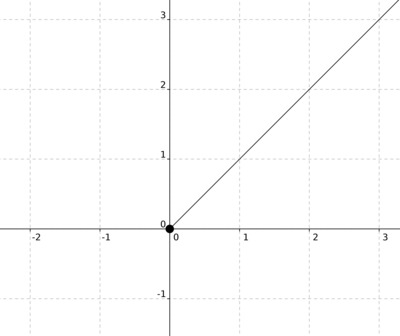# Graphs of Compositions

Alignments to Content Standards: F-BF.A.1.c

For each function in this task, assume the domain is the largest set of real numbers for which the function value is a real number.

Let $f$ be the function defined by $f(x)=x^2$. Let $g$ be the function defined by $g(x)=\sqrt{x}$.

1. Sketch the graph of $y=f(g(x))$ and explain your reasoning.
2. Sketch the graph of $y=g(f(x))$ and explain your reasoning.

## IM Commentary

This task addresses an important issue about inverse functions. In this case the function $f$ is the inverse of the function $g$ but $g$ is not the inverse of $f$ unless the domain of $f$ is restricted.

This task includes an experimental GeoGebra worksheet, with the intent that instructors might use it to more interactively demonstrate the relevant content material. The file should be considered a draft version, and feedback on it in the comment section is highly encouraged, both in terms of suggestions for improvement and for ideas on using it effectively. The file can be run via the free online application GeoGebra, or run locally if GeoGebra has been installed on a computer.

## Solution

1. We have $$f(g(x)) = (\sqrt{x})^2 = x.$$ The domain of $\sqrt{x}$ is all non-negative real numbers and so the graph starts at the point $(0,0)$ and then includes all positive values of $x$ in its domain:2. We have $$g(f(x)) = \sqrt{x^2}.$$ The square root $\sqrt{}$ takes non-negative real numbers as input and gives non-negative numbers as output. So $\sqrt{x^2} = x$ if $x$ is non-negative and $\sqrt{x^2} = -x$ if $x$ is negative. In other words $g(f(x)) = |x|,$ the absolute value function.